Function Repository Resource:

# DPSSWindow

Generate the zero-order discrete prolate spheroidal sequence window

Contributed by: Mariusz Jankowski
 ResourceFunction["DPSSWindow"][n] gives the order-0 discrete prolate spheroidal sequence (DPSS) window of length n. ResourceFunction["DPSSWindow"][n,α] uses the parameter α.

## Details and Options

The discrete prolate spheroidal sequence is also known as a Slepian sequence.
The discrete prolate spheroidal sequence of order 0 is a sequence known to have the maximum spectral concentration of any window sequence of the same length, that is based on prolate spheroidal wavefunctions. The very low side lobes of its frequency response make the DPSS useful as a data taper and as a finite impulse response (FIR) filter.
The parameter α is a positive number that defines the width of the main lobe, and typically takes values in the range 0α<π/2. It is by default set to π/4.

## Examples

### Basic Examples (2)

A DPSS window of length 21:

 In:=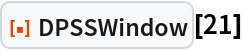Out=Visualize the window:

 In:=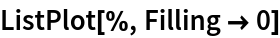Out=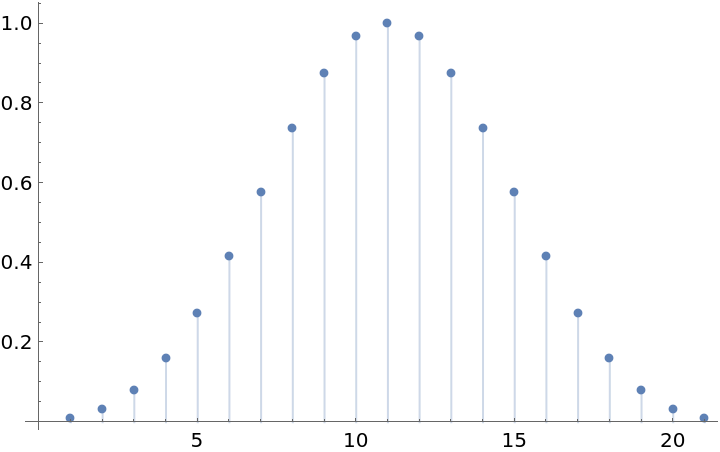A DPSS window with a specified parameter:

 In:=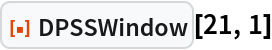Out=### Applications (2)

Create a lowpass FIR filter with cut-off frequency of π/5 and length 15:

 In:=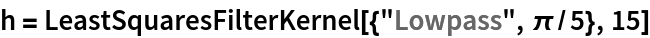Out=Apply a DPSS window to the filter to improve stop-band attenuation:

 In:=Out=Create a Log-magnitude plot of the power spectra of the two filters:

 In:=Out=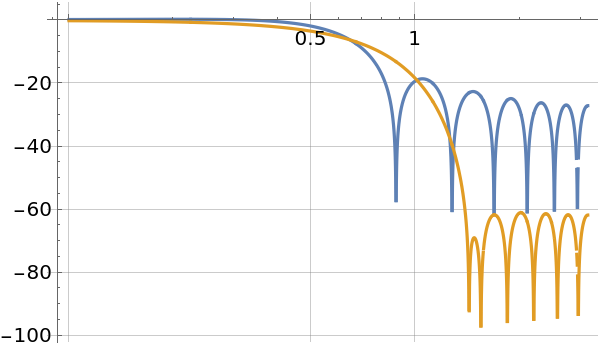Use the DPSS window to diminish the effect of signal partitioning when computing the spectrogram:

 In:=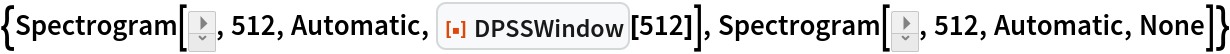Out=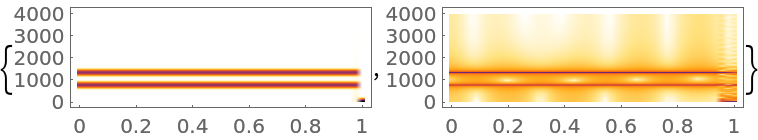### Properties and Relations (2)

DPSSWindow[n,0] is equivalent to the rectangular window:

 In:=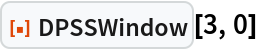Out=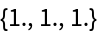The spectral concentration ratio of the DPSS window for length n and bandwidth -B<=ω<B:

 In:=Out=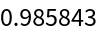Compare with a Blackman window:

 In:=Out=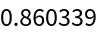## Publisher

Mariusz Jankowski

## Version History

• 1.0.0 – 15 February 2023